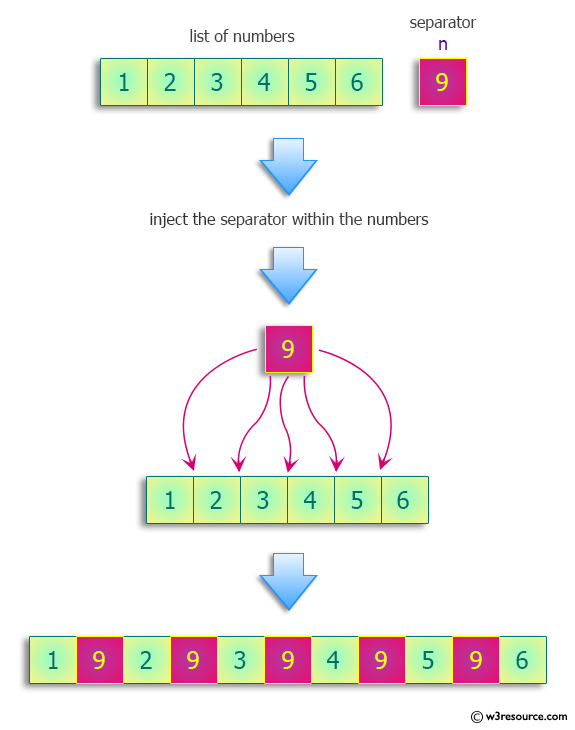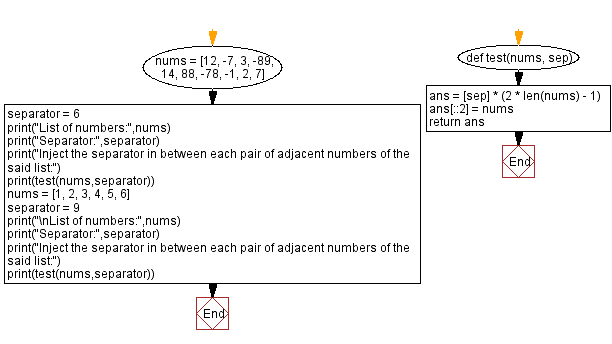﻿ Python: Inject a number in between each pair of adjacent numbers in a list of numbers - w3resource# Python: Inject a number in between each pair of adjacent numbers in a list of numbers

## Python Programming Puzzles: Exercise-71 with Solution

Given a list of numbers and a number to inject, write a Python program to create a list containing that number in between each pair of adjacent numbers.

```Input: [12, -7, 3, -89, 14, 88, -78, -1, 2, 7]
Separator: 6
Output:
[12, 6, -7, 6, 3, 6, -89, 6, 14, 6, 88, 6, -78, 6, -1, 6, 2, 6, 7]

Input: [1, 2, 3, 4, 5, 6]
Separator: 9
Output:
[1, 9, 2, 9, 3, 9, 4, 9, 5, 9, 6]
```

Pictorial Presentation:Sample Solution-1:

Python Code:

``````#License: https://bit.ly/3oLErEI

def test(nums, sep):
ans = [sep] * (2 * len(nums) - 1)
ans[::2] = nums
return ans
nums = [12, -7, 3, -89, 14, 88, -78, -1, 2, 7]
separator = 6
print("List of numbers:",nums)
print("Separator:",separator)
print("Inject the separator in between each pair of adjacent numbers of the said list:")
print(test(nums,separator))
nums = [1, 2, 3, 4, 5, 6]
separator = 9
print("\nList of numbers:",nums)
print("Separator:",separator)
print("Inject the separator in between each pair of adjacent numbers of the said list:")
print(test(nums,separator))
``````

Sample Output:

```List of numbers: [12, -7, 3, -89, 14, 88, -78, -1, 2, 7]
Separator: 6
Inject the separator in between each pair of adjacent numbers of the said list:
[12, 6, -7, 6, 3, 6, -89, 6, 14, 6, 88, 6, -78, 6, -1, 6, 2, 6, 7]

List of numbers: [1, 2, 3, 4, 5, 6]
Separator: 9
Inject the separator in between each pair of adjacent numbers of the said list:
[1, 9, 2, 9, 3, 9, 4, 9, 5, 9, 6]
```

Flowchart:## Visualize Python code execution:

The following tool visualize what the computer is doing step-by-step as it executes the said program:

Sample Solution-2:

Python Code:

``````#License: https://bit.ly/3oLErEI

def test(nums, sep):
result = []
for i in range(len(nums)):
if i == len(nums) - 1:
result.append(nums[i])
else:
result.append(nums[i])
result.append(sep)
return result

nums = [12, -7, 3, -89, 14, 88, -78, -1, 2, 7]
separator = 6
print("List of numbers:",nums)
print("Separator:",separator)
print("Inject the separator in between each pair of adjacent numbers of the said list:")
print(test(nums,separator))
nums = [1, 2, 3, 4, 5, 6]
separator = 9
print("\nList of numbers:",nums)
print("Separator:",separator)
print("Inject the separator in between each pair of adjacent numbers of the said list:")
print(test(nums,separator))
``````

Sample Output:

```List of numbers: [12, -7, 3, -89, 14, 88, -78, -1, 2, 7]
Separator: 6
Inject the separator in between each pair of adjacent numbers of the said list:
[12, 6, -7, 6, 3, 6, -89, 6, 14, 6, 88, 6, -78, 6, -1, 6, 2, 6, 7]

List of numbers: [1, 2, 3, 4, 5, 6]
Separator: 9
Inject the separator in between each pair of adjacent numbers of the said list:
[1, 9, 2, 9, 3, 9, 4, 9, 5, 9, 6]
```

## Visualize Python code execution:

The following tool visualize what the computer is doing step-by-step as it executes the said program:

Python Code Editor :

Have another way to solve this solution? Contribute your code (and comments) through Disqus.

What is the difficulty level of this exercise?

Test your Programming skills with w3resource's quiz.

﻿

## Python: Tips of the Day

Clamps num within the inclusive range specified by the boundary values x and y:

Example:

```def tips_clamp_num(num,x,y):
return max(min(num, max(x, y)), min(x, y))
print(tips_clamp_num(2, 4, 6))
print(tips_clamp_num(1, -1, -6))
```

Output:

```4
-1
```